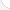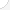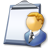Industrial engineersDescription: Design, develop, test, and evaluate integrated systems for managing industrial production processes, including human work factors, quality control, inventory control, logistics and material flow, cost analysis, and production coordination.Source: Bureau of Labor Statistics, U.S. Department of Labor, Occupational Outlook Handbook, 2008-09 Edition at http://www.bls.gov/OCO/Complete Job Profile: http://www.bls.gov/oco/ocos027.htmSalary: \$50,001 or more per yearComments:

There are 48 math topics Industrial engineers need to know.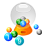Basic Math / Algebra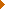FractionsDecimalsRatio and ProportionPercentCustomary MeasurementMetric MeasurementMeasurement ConversionBasic StatisticsStatistical GraphingPowers and RootsOther Number BasesNegative NumbersScientific NotationBasic Problem Solving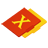First-Year AlgebraUsing FormulasLinear EquationsLinear InequalitiesOperations with PolynomialsCoordinate Graphing 2DQuadratic EquationsAlgebraic Representation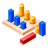GeometryBasic TerminologyAngle MeasurementCongruent TrianglesTriangle InequalitiesQuadrilateralsSimilarityPythagorean TheoremRight Triangle TrigonometryCirclesConstructionsAreaVolumeMake/Use 3D Drawings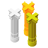Second-Year Algebra / TrigonometryFunctionsVariationImaginary NumbersLogarithmsAdvanced StatisticsPolar Coordinates/GraphsVectors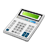Other TopicsBasic Calculator UseScientific Calculator UseComputer UseGroup Problem SolvingMental MathInductive/Deductive ReasoningMath Communications